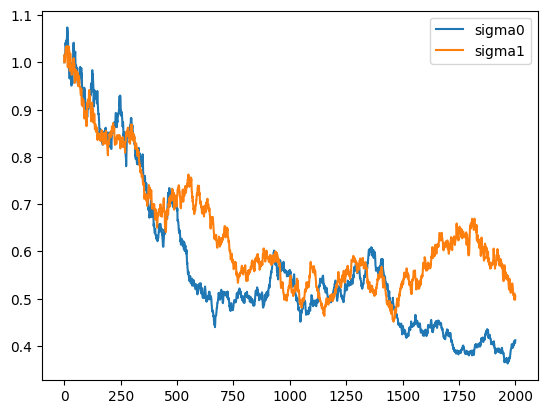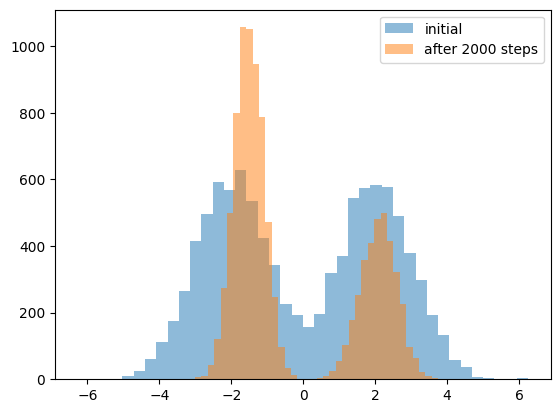# What happens when AI-generated data is used repeatedly for training

I read an interesting paper, The Curse of Recursion: Training on Generated Data Makes Models Forget, introduced in Import AI.

The content of the article pointed out that if data generated by a model is repeatedly used to train another model, information on the tail of the distribution is gradually lost, and the range of output becomes narrower. This should be especially important now that the results of generative AI are beginning to flood the Internet.

In the paper, they used theoretical and numerical analyses of Gaussian mixture as examples, and called this phenomenon model collapse. The numerical analysis seemed to be easy to do, so I checked it myself.

# Python implementation

What I want to do is to repeat the process of parameter fitting of one-dimensional Gaussian mixture distribution, generating new data from the fitted distribution, and fitting the distribution again based on the new data.

Specifically, given the training data for the $i$-th step, mix the following three to form the training data for the $i+1$-th step

• The training data for the $i$-th step
• A sample from the Gaussian mixture distribution fitted with the training data of the $i$-th
• Sample from the true distribution

For $i=0$, the samples are from the true Gaussian mixture distribution, and the ratio of the three data can be changed as desired.

from collections import namedtuple
import numpy as np
import matplotlib.pyplot as plt
from sklearn.mixture import GaussianMixture
from tqdm import tqdm

GaussianMixtureParams = namedtuple('GaussianMixtureParams', ('mus', 'sigmas', 'mixture_ratio'))

def sample_from_gaussian_mixture(gm_params, num_samples):
mus = np.array(gm_params.mus)
sigmas = np.array(gm_params.sigmas)

num_mixture = len(gm_params.mixture_ratio)
mixture_idx = np.random.choice(num_mixture, size=num_samples, p=gm_params.mixture_ratio)
standard_normal_noises = np.random.normal(size=num_samples)
samples = mus[mixture_idx] + standard_normal_noises * sigmas[mixture_idx]

return samples

def fit_gaussian_mixture(samples, n_components, random_state=0):
gm = GaussianMixture(n_components=n_components, random_state=random_state).fit(samples.reshape(-1,1))

mus = gm.means_.reshape(-1)
sorted_idx = np.argsort(mus)

gm_params = GaussianMixtureParams(
gm.means_.reshape(-1)[sorted_idx],
np.sqrt(gm.covariances_).reshape(-1)[sorted_idx],
gm.weights_[sorted_idx]
)

return gm_params

def sample_next_gen(gm_params_org, gm_params_fitted, samples_current_gen, ratio_org, ratio_fitted, num_samples):
num_samples_org = int(num_samples * ratio_org)
num_samples_fitted = int(num_samples * ratio_fitted)
num_samples_current = num_samples - num_samples_org - num_samples_fitted
if num_samples_current < 0:
raise ValueError("Invalid ratio")

samples_org = sample_from_gaussian_mixture(gm_params_org, num_samples_org)
samples_fitted = sample_from_gaussian_mixture(gm_params_fitted, num_samples_fitted)
samples_current = np.random.choice(samples_current_gen, size=num_samples_current, replace=False)

return np.concatenate([samples_org, samples_fitted, samples_current])


# Results

I ran a total of 2000 steps, sampling $N=10000$ each time. The true Gaussian mixture has two components and the parameters are

• $\boldsymbol \mu = (-2, 2)$
• $\boldsymbol \sigma = (1, 1)$
• mixture ratio = 1:1

For simplicity, only the data generated from the distribution fitted in the previous step is used for training

num_samples = 10000
num_gen = 2000

gm_params_org = GaussianMixtureParams([-2, 2], [1, 1], [0.5, 0.5])
samples = sample_from_gaussian_mixture(gm_params_org, num_samples)

for _ in tqdm(range(num_gen)):
gm_params_fitted = fit_gaussian_mixture(samples, 2)
samples = sample_next_gen(gm_params_org, gm_params_fitted, samples, 0.0, 1.0, num_samples)


Here is the plot of transition of the fitted varianceThe distribtuion gradually becomes narrower.

Comparing the initial distribution to that at the 2000th step, the mixing ratio also seems to be biased toward one of the mountains.However, when I repeated the process several times with different random number seeds, the fitted distribution sometimes went in a wider direction, and the speed of model collapse also changed depending on the mixing ratio of the three types of data. I am not sure how much of the results apply to real LLMs, etc.

The link to the notebook is here. Please let me know if there are any mistakes.